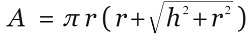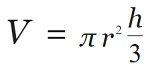# Rust program to calculate the surface area, the volume of the Cone

Given the radius and height of the Cone, we have to calculate the surface area, the volume of the Cone using the Rust program.
Submitted by Nidhi, on October 01, 2021

Problem Solution:

Here, we will read the height, radius from the user. Then we will calculate the surface area and volume of Cone and print the result.

The surface area of Cone:

To calculate the surface area of Cone (Right Circular Cone), the formula is:The volume of Cone:

To calculate the volume of Cone (Right Circular Cone), the formula is:Where,

• r : Radius of the Cone
• h : Height of the Cone

Program/Source Code:

The source code to calculate the surface area, volume of the Cone is given below. The given program is compiled and executed successfully.

```// Rust program to calculate
// the surface area, volume of Cone

use std::io;

fn main()
{
let mut height:f32  =0.0;

let mut area:f32    =0.0;
let mut volume:f32  =0.0;

let mut input1 = String::new();
let mut input2 = String::new();

println!("Enter height: ");
height = input1.trim().parse().expect("Not a valid number");

radius = input2.trim().parse().expect("Not a valid number");

volume = (1.0 / 3.0) * (3.14) * radius * radius * height;

println!("Volume of Cone 	  : {}", volume);
println!("Surface area of Cone: {}", area);
}
```

Output:

```RUN 1:
Enter height:
2.5
1.2
Volume of Cone    : 3.7680006
Surface area of Cone: 14.970586

RUN 2:
Enter height:
12.4
25.6
Volume of Cone    : 8505.699
Surface area of Cone: 4344.3564
```

Explanation:

Here, we read the height, radius from the user. Then we calculated the surface area, volume of the Cone and printed the result.